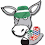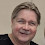## Tuesday, January 1, 2013

### NFL Picks Contest - Results and Standings

Here are the results and updated standings in the NFL Picks Contest.

Memphis MOJO shoots back into the lead, with Grange and lightning both moving up.

lightning- New England (W 28-0) = 38 pts.
TwoMinuteWarning- San Francisco (W 27-13) = 24 pts.
Grange95-  Denver (W 38-3) = 45 pts.
ohcowboy12go- No Pick = 0 pts.
Music City Momma- San Francisco (W 27-13) = 24 pts.
Memphis MOJO-  Denver (W 38-3) = 45 pts.
ggrouchie- San Francisco (W 27-13) = 24 pts.
Vegas Vic-  San Francisco (W 27-13) = 24 pts.
Captain Crunch- San Francisco (W 27-13) = 24 pts.

Here are the current standings;

Memphis MOJO - 40+19+22+13+16+18+24+68+22+17+45 = 304 pts.
Vegas Vic - 16+19+22+13+16+40+15+23+43+58+24 = 289
Grange95 - 20+19+22+13+14+40+24+23+43+17+45 = 280
TwoMinuteWarning - 13+19+22+16+31+18+19+23+31+58+24 = 274
Music City Momma - 17+19+24+5+17+34+24+24+43+17+24 =  248
lightning - 13+19+24+13+16+18+24+24+22+17+38 =228
Captain Crunch - 13+11+18+16+31+18+24-(2)+31+32+24 =216
grrouchie - 14+48+20+5+16+40+17+23-(28)+17+24 = 196
ohcowboy12go - 13+19+22+5+13+24+23-(7)+17+0 =129

Congratulations to Ninja MOJO for capturing the lead back!

Now, on to the playoffs!

1.1.2.